Theory and Modern Applications

# On the stability of ∗-derivations on Banach ∗-algebras

## Abstract

In the current paper, we study the stability and the superstability of -derivations associated with the Cauchy functional equation and the Jensen functional equation. We also prove the stability and the superstability of Jordan -derivations on Banach -algebras.

MSC:39B52, 47B47, 39B72, 47H10, 46H25.

## 1 Introduction

The basic problem of the stability of functional equations asks whether an approximate solution of the Cauchy functional equation $f\left(x+y\right)=f\left(x\right)+f\left(y\right)$ can be approximated by a solution of this equation . A functional equation is called stable if any approximately solution to the functional equation is near to a true solution of that functional equation, and is superstable if every approximately solution is an exact solution of it.

The study of stability problems for functional equations which had been proposed by Ulam  concerning the stability of group homomorphisms, affirmatively answered for Banach spaces by Hyers . The Hyers’ theorem was generalized by Aoki  and Bourgin  for additive mappings by considering an unbounded Cauchy difference. In , Th. M. Rassias succeeded in extending the result of the Hyers’ theorem by weakening the condition for the Cauchy difference controlled by ${\parallel a\parallel }^{r}+{\parallel b\parallel }^{r}$, $r\in \left[0,1\right)$ to be unbounded. Gǎvruta generalized the Rassias’ result in  for the unbounded Cauchy difference. And then, the stability problems of various functional equation have been extensively investigated by a number of authors and there are many interesting results concerning this problem (for instances,  and ). The stability of -derivations and of quadratic -derivations with the Cauchy functional equation and the Jensen functional equation on Banach -algebras was investigated in . Jang and Park  proved the superstability of -derivations and of quadratic -derivations on ${C}^{\ast }$-algebras. In , An, Cui, and Park investigated Jordan -derivations on ${C}^{\ast }$-algebras and Jordan -derivations on $J{C}^{\ast }$-algebras associated with a special functional inequality.

In 2003, Cǎdariu and Radu employed the fixed-point method to the investigation of the Jensen functional equation. They presented a short and a simple proof (different from the ‘direct method,’ initiated by Hyers in 1941) for the Cauchy functional equation  and for the quadratic functional equation . The Hyers-Ulam stability of the Jensen functional equation was studied by this method in . Also, this method is applied to prove the stability and the superstability for cubic and quartic functional equation under certain conditions on Banach algebras in [5, 6] (for the stability of ternary quadratic derivations on ternary Banach algebras and ${C}^{\ast }$-ternary rings, see ).

The stability and the superstability of homomorphisms on ${C}^{\ast }$-algebras by using the fixed point alternative (Theorem 2.1) were proved in . The Hyers-Ulam stability of -homomorphisms in unital ${C}^{\ast }$-algebras associated with the Trif functional equation, and of linear -derivations on unital ${C}^{\ast }$-algebras have earlier been established by Park and Hou in .

In this paper, we prove the stability of -derivations associated with the Cauchy functional equation and the Jensen functional equation on Banach -algebras. We also show that these functional equations under some mild conditions are superstable. We indicate a more accurate approximation than the results of Jang and Park which are obtained in . In fact, we obtain an extension and refinement of their results on Banach -algebras. So the condition of being ${C}^{\ast }$-algebra for A in  can be redundant.

## 2 Stability of ∗-derivations

Throughout this paper, assume that B is a Banach -algebra and that A is a Banach -subalgebra of B. A bounded $\mathbb{C}$-linear mapping $D:A\to B$ is said to be derivation on A if $D\left(ab\right)=D\left(a\right)\cdot b+a\cdot D\left(b\right)$ for all $a,b\in A$. In addition, if D satisfies the additional condition $D\left({a}^{\ast }\right)=D{\left(a\right)}^{\ast }$ for all $a\in A$, then it is called a -derivation.

Before proceeding to the main results, we will state the following theorem which is useful to our purpose (an extension of the result was given in ).

Theorem 2.1 (The fixed-point alternative )

Let$\left(\mathrm{\Omega },d\right)$be a complete generalized metric space and$\mathcal{T}:\mathrm{\Omega }\to \mathrm{\Omega }$be a mapping with Lipschitz constant$L<1$. Then, for each element$\alpha \in \mathrm{\Omega }$, either$d\left({\mathcal{T}}^{n}\alpha ,{\mathcal{T}}^{n+1}\alpha \right)=\mathrm{\infty }$for all$n\ge 0$, or there exists a natural number${n}_{0}$such that:

1. (i)

$d\left({\mathcal{T}}^{n}\alpha ,{\mathcal{T}}^{n+1}\alpha \right)<\mathrm{\infty }$ for all $n\ge {n}_{0}$;

2. (ii)

the sequence $\left\{{\mathcal{T}}^{n}\alpha \right\}$ is convergent to a fixed-point ${\beta }^{\ast }$ of $\mathcal{T}$;

3. (iii)

${\beta }^{\ast }$ is the unique fixed point of $\mathcal{T}$ in the set $\mathrm{\Lambda }=\left\{\beta \in \mathrm{\Omega }:d\left({\mathcal{T}}^{{n}_{0}}\alpha ,\beta \right)<\mathrm{\infty }\right\}$;

4. (iv)

$d\left(\beta ,{\beta }^{\ast }\right)\le \frac{1}{1-L}d\left(\beta ,\mathcal{T}\beta \right)$ for all $\beta \in \mathrm{\Lambda }$.

To achieve our maim in this section, we shall use the following lemma which is proved in .

Lemma 2.2 Let${n}_{0}\in \mathbb{N}$be a positive integer and let X, Y be complex vector spaces. Suppose that$f:X\to Y$is an additive mapping. Then f is$\mathbb{C}$-linear if and only if$f\left(\mu x\right)=\mu f\left(x\right)$for all x in X and μ in${\mathbb{T}}_{\frac{1}{{n}_{0}}}^{1}:=\left\{{e}^{i\theta }:0\le \theta \le \frac{2\pi }{{n}_{0}}\right\}$.

We establish the Hyers-Ulam stability of -derivations as follows:

Theorem 2.3 Let${n}_{0}\in \mathbb{N}$be fixed, $f:A\to B$a mapping with$f\left(0\right)=0$and let$\psi :{A}^{5}\to \left[0,\mathrm{\infty }\right)$be a function such that

$\parallel f\left(\mu a+b+xy\right)-\mu f\left(a\right)-f\left(b\right)-f\left(x\right)\cdot y-x\cdot f\left(y\right)-f\left({z}^{\ast }\right)-f{\left(z\right)}^{\ast }\parallel \le \psi \left(a,b,x,y,z\right)$
(1)

for all$\mu \in {\mathbb{T}}_{\frac{1}{{n}_{0}}}^{1}$and all$a,b,x,y,z\in A$. If there exists a constant$k\in \left(0,1\right)$, such that

$\psi \left(2a,2b,2x,2y,2z\right)\le 2k\psi \left(a,b,x,y,z\right)$
(2)

for all$a,b,x,y,z\in A$, then there exists a unique -derivation D on A satisfying

$\parallel f\left(a\right)-D\left(a\right)\parallel \le \frac{1}{2\left(1-k\right)}\stackrel{˜}{\psi }\left(a\right)\phantom{\rule{1em}{0ex}}\left(a\in A\right),$
(3)

where$\stackrel{˜}{\psi }\left(a\right)=\psi \left(a,a,0,0,0\right)$.

Proof First, we provide the conditions of Theorem 2.1. We consider the set

$\mathrm{\Omega }=\left\{g:A\to B|g\left(0\right)=0\right\}$

and define the mapping d on $\mathrm{\Omega }×\mathrm{\Omega }$ as follows:

$d\left({g}_{1},{g}_{2}\right):=inf\left\{c\in \left(0,\mathrm{\infty }\right):\parallel {g}_{1}\left(a\right)-{g}_{2}\left(a\right)\parallel \le c\stackrel{˜}{\psi }\left(a\right)\phantom{\rule{0.25em}{0ex}}\left(\mathrm{\forall }a\in A\right)\right\},$

if there exist such constant C, and $d\left({g}_{1},{g}_{2}\right)=\mathrm{\infty }$, otherwise. Similar to the proof of , Theorem 2.2], we can show that d is a generalized metric on Ω and the metric space $\left(\mathrm{\Omega },d\right)$ is complete. We define a mapping $\mathrm{\Psi }:\mathrm{\Omega }\to \mathrm{\Omega }$ by

$\mathrm{\Psi }\left(g\right)\left(a\right)=\frac{1}{2}g\left(2a\right)$
(4)

for all $a\in A$. We show that Ψ is strictly contractive on Ω. Given ${g}_{1},{g}_{2}\in \mathrm{\Omega }$, let $c\in \left(0,\mathrm{\infty }\right)$ be an arbitrary constant with $d\left({g}_{1},{g}_{2}\right)\le c$. It means that

$\parallel {g}_{1}\left(a\right)-{g}_{2}\left(a\right)\parallel \le c\stackrel{˜}{\psi }\left(a\right)$
(5)

for all $a\in A$. Substituting a by 2a in the inequality (5) and using (2) and (4), we get

$\parallel \mathrm{\Psi }{g}_{1}\left(a\right)-\mathrm{\Psi }{g}_{2}\left(a\right)\parallel =\frac{1}{2}\parallel {g}_{1}\left(2a\right)-{g}_{2}\left(2a\right)\parallel \le \frac{1}{2}c\stackrel{˜}{\psi }\left(2a\right)\le ck\stackrel{˜}{\psi }\left(a\right)$

for all $a\in A$. Then $d\left(\mathrm{\Psi }{g}_{1},\mathrm{\Psi }{g}_{2}\left(a\right)\right)\le ck$. This shows that

$d\left(\mathrm{\Psi }{g}_{1},\mathrm{\Psi }{g}_{2}\right)\le Kd\left({g}_{1},{g}_{2}\right)$

for all ${g}_{1},{g}_{2}\in \mathrm{\Omega }$. To achieve inequality (3), we prove that $d\left(\mathrm{\Psi }f,f\right)<\mathrm{\infty }$. Putting $a=b$, $x=y=z=0$, and $\mu =1$ (in fact $1\in {\mathbb{T}}_{\frac{1}{n}}^{1}$ for all $n\in \mathbb{N}$) in (1), we obtain

$\parallel f\left(2a\right)-2f\left(a\right)\parallel \le \stackrel{˜}{\psi }\left(a\right)$

for all $a\in A$. Hence,

$\parallel \frac{1}{2}f\left(2a\right)-f\left(a\right)\parallel \le \frac{1}{2}\stackrel{˜}{\psi }\left(a\right)$
(6)

for all $a\in A$. We conclude from (6) that $d\left(\mathrm{\Psi }f,f\right)\le \frac{1}{2}$. It follows from Theorem 2.1 that $d\left({\mathcal{J}}^{n}g,{\mathcal{J}}^{n+1}g\right)<\mathrm{\infty }$ for all $n\ge 0$, and thus in this theorem we have ${n}_{0}=0$. Therefore, the parts (iii) and (iv) of Theorem 2.1 hold on the whole Ω. Hence, there exists a unique mapping $D:A\to B$ such that D is a fixed point of T and that ${\mathrm{\Psi }}^{n}f\to D$ as $n\to \mathrm{\infty }$. Thus,

$\underset{n\to \mathrm{\infty }}{lim}\frac{f\left({2}^{n}a\right)}{{2}^{n}}=D\left(a\right)$
(7)

for all $a\in A$, and so

$d\left(f,D\right)\le \frac{1}{1-k}d\left(\mathrm{\Psi }f,f\right)\le \frac{1}{2\left(1-k\right)}.$

The above inequality shows that (3) is true for all $a\in A$. It follows from (2) that

$\underset{n\to \mathrm{\infty }}{lim}\frac{\psi \left({2}^{n}a,{2}^{n}b,{2}^{n}x,{2}^{n}y,{2}^{n}z\right)}{{2}^{n}}=0.$
(8)

Now, we replace a by ${2}^{n}a$ and put $b=x=y=z=0$ in (1). We divide both sides of the resulting inequality by ${2}^{n}$, and let n tend to infinity. It follows from (1), (7), and (8) that

$D\left(\mu a\right)=\mu D\left(a\right)$

for all $a\in A$ and all $\mu \in {\mathbb{T}}_{\frac{1}{{n}_{0}}}^{1}$. Now, by Lemma 2.2, D is $\mathbb{C}$-linear. Replacing x, y by ${2}^{n}x$, ${2}^{n}y$, respectively, and putting $a=b=z=0$ in (1), we have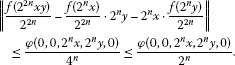Taking the limit as n tend to infinity, we get $D\left(xy\right)=D\left(x\right)\cdot y+x\cdot D\left(y\right)$ for all $x,y\in A$. If we put $a=b=x=y=0$ and substitute z by ${2}^{n}z$ in (1) and we divide the both sides of the obtained inequality by ${2}^{n}$, then we get

$\parallel \frac{f\left({2}^{n}{z}^{\ast }\right)}{{2}^{n}}-\frac{f{\left({2}^{n}z\right)}^{\ast }}{{2}^{n}}\parallel \le \frac{\phi \left(0,0,0,0,{2}^{n}z\right)}{{2}^{n}}$
(9)

for all $z\in A$. Passing to the limit as $n\to \mathrm{\infty }$ in (9), we conclude that $D\left({z}^{\ast }\right)=D{\left(z\right)}^{\ast }$ for all $z\in A$. Thus, D is a -derivation. □

Corollary 2.4 Let${n}_{0}\in \mathbb{N}$be fixed, $r\in \left(0,1\right)$, and let$f:A\to B$be mappings with$f\left(0\right)=0$such that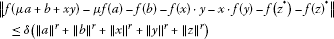for all$\mu \in {\mathbb{T}}_{\frac{1}{{n}_{0}}}^{1}$and all$a,b,x,y,z\in A$. Then there exists a unique -derivation D on A satisfying

$\parallel f\left(a\right)-D\left(a\right)\parallel \le \frac{2\delta }{2-{2}^{r}}{\parallel a\parallel }^{r}$

for all$a\in A$.

Proof The proof follows from Theorem 2.3 by taking

$\psi \left(a,b,x,y,z\right)=\delta \left({\parallel a\parallel }^{r}+{\parallel b\parallel }^{r}+{\parallel x\parallel }^{r}+{\parallel y\parallel }^{r}+{\parallel z\parallel }^{r}\right)$

for all $a,b,x,y,z\in A$ and $k={2}^{r-1}$. □

In the following corollaries, we show that under some conditions the superstability for the inequality (1) is valid.

Corollary 2.5 Let$f:A\to B$be an additive mapping satisfying (1) and$\psi :{A}^{5}\to \left[0,\mathrm{\infty }\right)$be a function satisfying (2). Then f is a -derivation.

Proof It follows immediately from additivity of f that $f\left(0\right)=0$. Thus, $f\left({2}^{n}a\right)={2}^{n}f\left(a\right)$ for all $a\in A$. Now, by the proof of Theorem 2.3, f is a -derivation. □

Corollary 2.6 Let${r}_{j}$ ($1\le j\le 5$), δ be nonnegative real numbers with$0<{\sum }_{j=1}^{5}{r}_{j}\ne 1$and let$f:A\to B$be a mapping with$f\left(0\right)=0$such that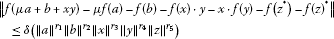(10)

for all$\mu \in {\mathbb{T}}_{\frac{1}{{n}_{0}}}^{1}$and all$a,b,x,y,z\in A$. Then f is a -derivation on A.

Proof If we put $a=b=x=y=z=0$ and $\mu =1$ in (10), we get $f\left(0\right)=0$. Again, putting $a=b$, $x=y=z=0$, and $\mu =1$ in (10), we conclude that $f\left(2a\right)=2f\left(a\right)$, and by induction we have $f\left(a\right)=\frac{f\left({2}^{n}a\right)}{{2}^{n}}$ for all $a\in A$ and $n\in \mathbb{N}$. Now, we can obtain the desired result by Theorem 2.3. □

A bounded $\mathbb{C}$-linear mapping $D:A\to A$ is said to be Jordan derivation on A if $D\left({a}^{2}\right)=D\left(a\right)\cdot a+a\cdot D\left(a\right)$ for all $a,b\in A$. Note that the mapping $x↦ax-xa$, where a is a fixed element in A, is a Jordan -derivation. For the first time, Jordan -derivations were introduced in [23, 24] and the structure of such derivations has investigated in . The reason for introducing these mappings was the fact that the problem of representing quadratic forms by sesquilinear ones is closely connected with the structure of Jordan -derivations.

The next theorem is in analogy with Theorem 2.3 for Jordan -derivations. Since the proof is similar, it is omitted.

Theorem 2.7 Let${n}_{0}\in \mathbb{N}$be fixed, $f:A\to A$a mapping with$f\left(0\right)=0$and let$\psi :{A}^{3}\to \left[0,\mathrm{\infty }\right)$be a function such that

$\parallel f\left(\mu a+b+{c}^{2}\right)-\mu f\left(a\right)-f\left(b\right)-f\left(c\right)\cdot {c}^{\ast }-c\cdot f\left(c\right)\parallel \le \psi \left(a,b,c\right)$
(11)

for all$\mu \in {\mathbb{T}}_{\frac{1}{{n}_{0}}}^{1}$and all$a,b,c\in A$. If there exists a constant$k\in \left(0,1\right)$, such that

$\psi \left(2a,2b,2c\right)\le 2k\psi \left(a,b,c\right)$
(12)

for all$a,b,c\in A$, then there exists a unique Jordan -derivation D on A satisfying

$\parallel f\left(a\right)-D\left(a\right)\parallel \le \frac{1}{2-2k}\psi \left(a,a,0\right)$

for all$a\in A$.

The following corollaries are analogous to Corollaries 2.4, 2.5, and 2.6, respectively. The proofs are similar and so we omit them.

Corollary 2.8 Let${n}_{0}\in \mathbb{N}$be fixed, $r\in \left(0,1\right)$, and let$f:A\to A$be mappings with$f\left(0\right)=0$such that

$\parallel f\left(\mu a+b+{c}^{2}\right)-\mu f\left(a\right)-f\left(b\right)-f\left(c\right)\cdot {c}^{\ast }-c\cdot f\left(c\right)\parallel \le \delta \left({\parallel a\parallel }^{r}+{\parallel b\parallel }^{r}+{\parallel c\parallel }^{r}\right)$

for all$\mu \in {\mathbb{T}}_{\frac{1}{{n}_{0}}}^{1}$and all$a,b,c,d\in A$. Then there exists a unique Jordan -derivation D on A satisfying

$\parallel f\left(a\right)-D\left(a\right)\parallel \le \frac{2\delta }{2-{2}^{r}}{\parallel a\parallel }^{r}$

for all$a\in A$.

Corollary 2.9 Let$f:A\to A$be an additive mapping satisfying (11) and let$\psi :{A}^{3}\to \left[0,\mathrm{\infty }\right)$be a function satisfying (12). Then f is a Jordan -derivation.

Corollary 2.10 Let${r}_{j}$ ($1\le j\le 3$), δ be nonnegative real numbers with$0<{\sum }_{j=1}^{3}{r}_{j}\ne 1$and let$f:A\to B$be a mapping with$f\left(0\right)=0$such that

$\parallel f\left(\mu a+b+{c}^{2}\right)-\mu f\left(a\right)-f\left(b\right)-f\left(c\right)\cdot {c}^{\ast }-c\cdot f\left(c\right)\parallel \le \delta \left({\parallel a\parallel }^{{r}_{1}}{\parallel b\parallel }^{{r}_{2}}{\parallel c\parallel }^{{r}_{3}}\right)$

for all$\mu \in {\mathbb{T}}_{\frac{1}{{n}_{0}}}^{1}$and for all$a,b,c\in A$. Then f is a Jordan -derivation on A.

## 3 Stability of ∗-derivations associated with the Jensen functional equation

In this section, we investigate the stability and the superstability of -derivations associated with the Jensen functional equation in Banach -algebra.

Theorem 3.1 Let${n}_{0}\in \mathbb{N}$be fixed, $f:A\to B$a mapping with$f\left(0\right)=0$and let$\varphi :{A}^{5}\to \left[0,\mathrm{\infty }\right)$be a function such that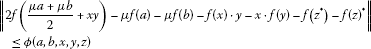(13)

for all$\mu \in {\mathbb{T}}_{\frac{1}{{n}_{0}}}^{1}$and all$a,b,x,y,z\in A$. If there exists a constant$k\in \left(0,1\right)$, such that

$\varphi \left(2a,2b,2x,2y,2z\right)\le 2k\varphi \left(a,b,x,y,z\right)$
(14)

for all$a,b,x,y,z\in A$, then there exists a unique -derivation D on A satisfying

$\parallel f\left(a\right)-D\left(a\right)\parallel \le \frac{k}{1-k}\stackrel{˜}{\varphi }\left(a\right)\phantom{\rule{1em}{0ex}}\left(a\in A\right),$
(15)

where$\stackrel{˜}{\varphi }\left(a\right)=\varphi \left(a,0,0,0,0\right)$.

Proof Similar to the proof of Theorem 2.3, we consider the set

$\mathrm{\Omega }=\left\{g:A\to g|g\left(0\right)=0\right\}$

and define the mapping d on $\mathrm{\Omega }×\mathrm{\Omega }$ as follows:

$d\left({g}_{1},{g}_{2}\right):=inf\left\{c\in \left(0,\mathrm{\infty }\right):\parallel {g}_{1}\left(a\right)-{g}_{2}\left(a\right)\parallel \le c\stackrel{˜}{\varphi }\left(a\right),\mathrm{\forall }a\in A\right\},$

if there exist such constant C, and $d\left({g}_{1},{g}_{2}\right)=\mathrm{\infty }$, otherwise. The metric space $\left(\mathrm{\Omega },d\right)$ is complete and also the mapping $\mathrm{\Phi }:\mathrm{\Omega }\to \mathrm{\Omega }$ defined by

$\mathrm{\Phi }\left(g\right)\left(a\right)=\frac{1}{2}g\left(2a\right)\phantom{\rule{1em}{0ex}}\left(a\in A\right)$
(16)

is strictly contractive on Ω. Putting $\mu =1$ and $b=x=y=z=0$ in (13), we obtain

$\parallel 2f\left(\frac{a}{2}\right)-f\left(a\right)\parallel \le \stackrel{˜}{\varphi }\left(a\right)$

for all $a\in A$. By (14), we get

$\parallel \frac{1}{2}f\left(2a\right)-f\left(a\right)\parallel \le \frac{1}{2}\stackrel{˜}{\varphi }\left(2a\right)\le k\stackrel{˜}{\varphi }\left(a\right)$
(17)

for all $a\in A$. So by (17), we have $d\left(\mathrm{\Phi }f,f\right)\le k$. Since the parts (iii) and (iv) of Theorem 2.1 hold on the whole Ω, there exists a unique mapping $D:A\to B$ such that D is a fixed point of Φ such that

$\underset{n\to \mathrm{\infty }}{lim}\frac{f\left({2}^{n}a\right)}{{2}^{n}}=D\left(a\right)$
(18)

for all $a\in A$, and so

$d\left(f,D\right)\le \frac{1}{1-k}d\left(\mathrm{\Phi }f,f\right)\le \frac{k}{1-k}.$

So (15) holds for all $a\in A$. It follows from (14) that

$\underset{n\to \mathrm{\infty }}{lim}\frac{\psi \left({2}^{n}a,{2}^{n}b,{2}^{n}x,{2}^{n}y,{2}^{n}z\right)}{{2}^{n}}=0.$
(19)

Letting $a=b$ and putting $x=y=z=0$ in (13), we have

$\parallel f\left(\mu a\right)-\mu f\left(a\right)\parallel \le \frac{1}{2}\varphi \left(a,a,0,0,0\right).$
(20)

Now, replacing a by ${2}^{n}a$ in (20) and dividing both sides of the resulting inequality by ${2}^{n}$, and letting $n\to \mathrm{\infty }$, by (13), (18), and (19), we have

$D\left(\mu a\right)=\mu D\left(a\right)$

for all $a\in A$ and all $\mu \in {\mathbb{T}}_{\frac{1}{{n}_{0}}}^{1}$. By Lemma 2.2, D is $\mathbb{C}$-linear.

The rest of the proof is similar to the proof of Theorem 2.3. □

Corollary 3.2 Let${n}_{0}\in \mathbb{N}$be fixed, $r\in \left(0,1\right)$, and let$f:A\to B$be mappings with$f\left(0\right)=0$such that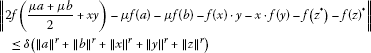for all$\mu \in {\mathbb{T}}_{\frac{1}{{n}_{0}}}^{1}$and all$a,b,x,y,z\in A$. Then there exists a unique -derivation D on A satisfying

$\parallel f\left(a\right)-D\left(a\right)\parallel \le \frac{{2}^{r}\delta }{2-{2}^{r}}{\parallel a\parallel }^{r}$

for all$a\in A$.

Proof The proof follows from Theorem 3.1 by taking $\varphi \left(a,b,x,y,z\right)=\delta \left({\parallel a\parallel }^{r}+{\parallel b\parallel }^{r}+{\parallel x\parallel }^{r}+{\parallel y\parallel }^{r}+{\parallel z\parallel }^{r}\right)$ for all $a,b,x,y,z\in A$ and $k={2}^{r-1}$. □

In the following corollary, we show that when f is an additive mapping, the superstability for the inequality (13) holds.

Corollary 3.3 Let$f:A\to B$be an additive mapping satisfying (13) and let$\psi :{A}^{5}\to \left[0,\mathrm{\infty }\right)$be a function satisfying (14). Then f is a -derivation.

Proof The proof is similar to the proof of Corollary 2.5. □

Corollary 3.4 Let $f:A\to B$ be a mapping with $f\left(0\right)=0$ such that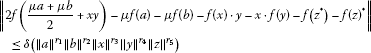(21)

for all$\mu \in {\mathbb{T}}_{\frac{1}{{n}_{0}}}^{1}$and all$a,b,x,y,z\in A$. If${r}_{j}$ ($1\le j\le 5$), δ are nonnegative real numbers such that$0<{\sum }_{j=1}^{5}{r}_{j}\ne 1$, then f is a -derivation.

Proof Putting $a=b=x=y=z=0$ and $\mu =1$ in (21), we obtain $f\left(0\right)=0$. Replacing a by 2a and setting $b=x=y=z=0$ and $\mu =1$ in (21), we have $f\left(2a\right)=2f\left(a\right)$, and thus $f\left(a\right)=\frac{f\left({2}^{n}a\right)}{{2}^{n}}$ for all $a\in A$ and $n\in \mathbb{N}$. Now, Theorem 3.1 shows that f is a -derivation on A. □

Theorem 3.5 Let${n}_{0}$be a fixed natural number, $f:A\to B$a mapping with$f\left(0\right)=0$and let$\varphi :{A}^{5}\to \left[0,\mathrm{\infty }\right)$be a function such that(22)

for all$\mu \in {\mathbb{T}}_{\frac{1}{{n}_{0}}}^{1}$and all$a,b,x,y,z\in A$. If there exists a constant$k\in \left(0,1\right)$such that

$\varphi \left(3a,3b,3x,3y,3z\right)\le 3k\varphi \left(a,b,x,y,z\right)$
(23)

for all$a,b,x,y,z\in A$, then there exists a unique -derivation D on A satisfying

$\parallel f\left(a\right)-D\left(a\right)\parallel \le \frac{1}{3\left(1-k\right)}\left({\stackrel{˜}{\varphi }}_{1}\left(a\right)+{\stackrel{˜}{\varphi }}_{2}\left(a\right)\right)\phantom{\rule{1em}{0ex}}\left(a\in A\right),$
(24)

where${\stackrel{˜}{\varphi }}_{1}\left(a\right)=\varphi \left(a,-a,0,0,0\right)$and${\stackrel{˜}{\varphi }}_{2}\left(a\right)=\varphi \left(-a,3a,0,0,0\right)$.

Proof Suppose that the set Ω as in the proof of Theorem 3.1. We introduce the generalized metric on Ω as follows:

if there exists such constant c, and $d\left(g,h\right)=\mathrm{\infty }$, otherwise. One can prove that the metric space $\left(\mathrm{\Omega },d\right)$ is complete. Define the mapping $\mathrm{\Phi }:\mathrm{\Omega }\to \mathrm{\Omega }$via

$\mathrm{\Phi }\left(g\right)\left(a\right)=\frac{1}{3}g\left(3a\right)\phantom{\rule{1em}{0ex}}\left(a\in A\right).$
(25)

Given $g,h\in \mathrm{\Omega }$, let $c\in \left(0,\mathrm{\infty }\right)$ be an arbitrary constant with $d\left(g,h\right)\le c$, that is,

$\parallel g\left(a\right)-h\left(a\right)\parallel \le c\left({\stackrel{˜}{\varphi }}_{1}\left(a\right)+{\stackrel{˜}{\varphi }}_{2}\left(a\right)\right)$
(26)

for all $a\in A$. If we substitute a by 3a in the inequality (26) and use from (23) and (25), we have

$\parallel \mathrm{\Phi }g\left(a\right)-\mathrm{\Phi }h\left(a\right)\parallel =\frac{1}{3}\parallel g\left(3a\right)-h\left(3a\right)\parallel \le \frac{1}{3}c\left({\stackrel{˜}{\varphi }}_{1}\left(3a\right)+{\stackrel{˜}{\varphi }}_{2}\left(3a\right)\right)\le ck\left({\stackrel{˜}{\varphi }}_{1}\left(a\right)+{\stackrel{˜}{\varphi }}_{2}\left(a\right)\right)$

for all $a\in A$. Thus, $d\left(\mathrm{\Psi }{g}_{1},\mathrm{\Psi }{g}_{2}\left(a\right)\right)\le ck$. This shows that Ψ is strictly contractive on Ω. Letting $\mu =1$, $b=-a$ and $x=y=z=0$ in (22), we have

$\parallel -f\left(a\right)-f\left(-a\right)\parallel \le {\stackrel{˜}{\varphi }}_{1}\left(a\right)$

for all $a\in A$. Putting $\mu =1$, $x=y=z=0$ and replacing a, b by 3a, −a in (22), respectively, we get

$\parallel 2f\left(a\right)-f\left(-a\right)-f\left(3a\right)\parallel \le {\stackrel{˜}{\varphi }}_{2}\left(a\right)$

for all $a\in A$. Hence,

$\begin{array}{rcl}\parallel \frac{1}{3}f\left(3a\right)-f\left(a\right)\parallel & \le & \frac{1}{3}\left(\parallel -f\left(a\right)-f\left(-a\right)\parallel +\parallel 2f\left(a\right)-f\left(-a\right)-f\left(3a\right)\parallel \right)\\ \le & \frac{1}{3}\left[{\stackrel{˜}{\varphi }}_{1}\left(a\right)+{\stackrel{˜}{\varphi }}_{2}\left(a\right)\right]\end{array}$

for all $a\in A$. The above inequality shows that $d\left(\mathrm{\Phi }f,f\right)\le \frac{1}{3}$. Now, by Theorem 2.1, there exists a unique mapping $D:A\to B$ such that D is a fixed point of Φ such that

$D\left(a\right)=\underset{n\to \mathrm{\infty }}{lim}\frac{f\left({3}^{n}a\right)}{{3}^{n}}$
(27)

for all $a\in A$, and thus $d\left(f,D\right)\le \frac{1}{1-k}d\left(\mathrm{\Phi }f,f\right)\le \frac{1}{3\left(1-k\right)}$. So we proved the inequality (24). By the inequality (23), we get

$\underset{n\to \mathrm{\infty }}{lim}\frac{\psi \left({3}^{n}a,{3}^{n}b,{3}^{n}x,{3}^{n}y,{3}^{n}z\right)}{{3}^{n}}=0.$
(28)

Letting $a=b$ and putting $x=y=z=0$ in (22), we have

$\parallel f\left(\mu a\right)-\mu f\left(a\right)\parallel \le {\stackrel{˜}{\varphi }}_{3}\left(a\right),$
(29)

where ${\stackrel{˜}{\varphi }}_{3}\left(a\right)=\varphi \left(a,a,0,0,0\right)$. If we replace a by ${3}^{n}a$ in (29) and divide both sides of the resulting inequality by ${3}^{n}$, we have

$\frac{1}{{3}^{n}}\parallel f\left({3}^{n}\mu a\right)-\mu f\left({3}^{n}a\right)\parallel \le \frac{1}{{3}^{n}}{\stackrel{˜}{\varphi }}_{3}\left({3}^{n}a\right).$

Let n tend to infinity. It follows from (22), (27), and (28) that $D\left(\mu a\right)=\mu D\left(a\right)$ for all $a\in A$ and all $\mu \in {\mathbb{T}}_{\frac{1}{{n}_{0}}}^{1}$. Similar to the above and again from (22) by applying (27) and (28), we can prove that $2D\left(\frac{a+b}{2}\right)=D\left(a\right)+D\left(b\right)$ for all $a,b\in A$. Since $f\left(0\right)=0$, we have $D\left(0\right)=0$. Therefore, $2D\left(\frac{a}{2}\right)=D\left(a\right)$ for every $a\in A$, and thus

$D\left(a\right)+D\left(b\right)=2D\left(\frac{a+b}{2}\right)=D\left(a+b\right),$

for all $a,b\in A$. Now, Lemma 2.2 shows that D is $\mathbb{C}$-linear. The rest of the proof is similar to the proof of Theorem 2.3. □

## References

1. An J, Cui J, Park C:Jordan -derivations on ${C}^{\ast }$-algebras and $J{C}^{\ast }$-algebras. Abstr. Appl. Anal. 2008., 2008: Article ID 410437

2. Aoki T: On the stability of the linear transformation in Banach spaces. J. Math. Soc. Jpn. 1950, 2: 64–66. 10.2969/jmsj/00210064

3. Brešar M, Zalar B: On the structure of Jordan -derivations. Colloq. Math. 1992, LXIII: 163–171.

4. Bodaghi A, Alias IA:Approximate ternary quadratic derivations on ternary Banach algebras and ${C}^{\ast }$-ternary rings. Adv. Differ. Equ. 2012., 2012: Article ID 11

5. Bodaghi A, Alias IA, Eshaghi Gordji M: On the stability of quadratic double centralizers and quadratic multipliers: a fixed point approach. J. Inequal. Appl. 2011., 2011: Article ID 957541

6. Bodaghi A, Alias IA, Ghahramani MH: Approximately cubic functional equations and cubic multipliers. J. Inequal. Appl. 2011., 2011: Article ID 53

7. Bodaghi A, Alias IA, Ghahramani MH: Ulam stability of a quartic functional equation. Abstr. Appl. Anal. 2012., 2012: Article ID 232630. doi:10.1155/2012/232630

8. Bourgin DG: Classes of transformations and bordering transformations. Bull. Am. Math. Soc. 1951, 57: 223–237. 10.1090/S0002-9904-1951-09511-7

9. Cădariu L, Radu V: Fixed points and the stability of quadratic functional equations. An. Univ. Timiş., Ser. Mat.-Inf. 2003, 41: 25–48.

10. Cădariu L, Radu V: On the stability of the Cauchy functional equation: a fixed point approach. Grazer Math. Ber. 2004, 346: 43–52.

11. Diaz JB, Margolis B: A fixed point theorem of the alternative for contractions on a generalized complete metric space. Bull. Am. Math. Soc. 1968, 74: 305–309. 10.1090/S0002-9904-1968-11933-0

12. Eshaghi Gordji M, Bodaghi A: On the Hyers-Ulam-Rasias stability problem for quadratic functional equations. East J. Approx. 2010, 16: 123–130.

13. Eshaghi Gordji M, Bodaghi A, Park C: A fixed point approach to the stability of double Jordan centralizers and Jordan multipliers on Banach algebras. Politehn. Univ. Bucharest Sci. Bull. Ser. A 2011, 73: 65–73.

14. Eshaghi Gordji M, Kaboli Gharetapeh S, Bidkham M, Karimi T, Aghaei M:Almost homomorphism between unital ${C}^{\ast }$-algebras: a fixed point approach. Anal. Theory Appl. 2011, 27(4):320–331. 10.1007/s10496-011-0320-3

15. Gǎvruta P: A generalization of the Hyers-Ulam-Rassias stability of approximately additive mappings. J. Math. Anal. Appl. 1994, 184: 431–436. 10.1006/jmaa.1994.1211

16. Hyers DH: On the stability of the linear functional equation. Proc. Natl. Acad. Sci. USA 1941, 27: 222–224. 10.1073/pnas.27.4.222

17. Hyers DH, Isac G, Rassias TM: Stability of Functional Equations in Several Variables. Birkhäuser, Basel; 1998.

18. Jang S, Park C:Approximate -derivations and approximate quadratic -derivations on ${C}^{\ast }$-algebras. J. Inequal. Appl. 2011., 2011: Article ID 55

19. Park C: Fixed points and Hyers-Ulam-Rassias stability of Cauchy-Jensen functional equations in Banach algebras. Fixed Point Theory Appl. 2007., 2007: Article ID 50175

20. Park C, Hou J:Homomorphisms between ${C}^{\ast }$-algebras associated with the Trif functional equation and linear derivations on ${C}^{\ast }$-algebras. J. Korean Math. Soc. 2004, 41: 461–477.

21. Pólya G, Szegö G I. In Aufgaben und Lehrsätze aus der Analysis. Springer, Berlin; 1925.

22. Rassias TM: On the stability of the linear mapping in Banach spaces. Proc. Am. Math. Soc. 1978, 72: 297–300. 10.1090/S0002-9939-1978-0507327-1

23. Šemrl P: On Jordan -derivations and an application. Colloq. Math. 1990, 59: 241–251.

24. Šemrl P: Quadratic functionals and Jordan -derivations. Stud. Math. 1991, 97: 157–163.

25. Turinici M: Sequentially iterative processes and applications to Volterra functional equations. Ann. Univ. Mariae Curie-Skl̄odowska, Sect. A 1978, 32: 127–134.

26. Ulam SM Science Ed. In Problems in Modern Mathematics. Wiley, New York; 1940. Chapter VI

## Acknowledgement

The authors would like to thank the referee for careful reading of the paper and giving some useful suggestions.

## Author information

Authors

### Corresponding author

Correspondence to Abasalt Bodaghi.

### Competing interests

The authors declare that they have no competing interests.

### Authors’ contributions

The study presented here was carried out in collaboration between all authors. AB suggested to write the current article. All authors read and approved the final manuscript.

## Rights and permissions

Reprints and Permissions

Park, C., Bodaghi, A. On the stability of -derivations on Banach -algebras. Adv Differ Equ 2012, 138 (2012). https://doi.org/10.1186/1687-1847-2012-138

• Accepted:

• Published:

• DOI: https://doi.org/10.1186/1687-1847-2012-138

### Keywords

• -derivation
• Banach -algebra
• Jordan -derivation
• stability
• superstability